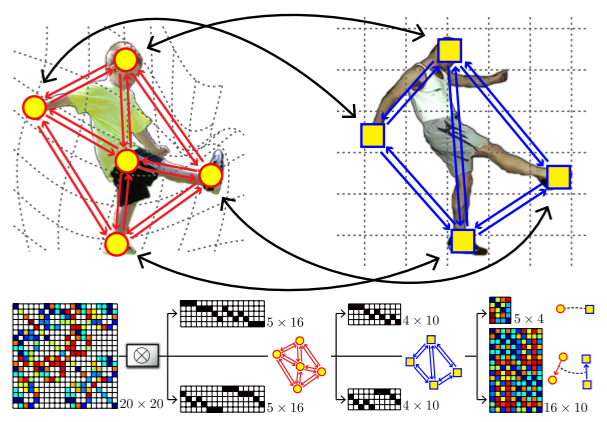# src.factorize_graph_matching.construct_aff_mat¶

src.factorize_graph_matching.construct_aff_mat(Ke: torch.Tensor, Kp: torch.Tensor, KroG: src.sparse_torch.csx_matrix.CSRMatrix3d, KroH: src.sparse_torch.csx_matrix.CSCMatrix3d, KroGt: Optional[src.sparse_torch.csx_matrix.CSRMatrix3d] = None, KroHt: Optional[src.sparse_torch.csx_matrix.CSCMatrix3d] = None) torch.Tensor[source]

Construct the complete affinity matrix with edge-wise affinity matrix $$\mathbf{K}_e$$, node-wise matrix $$\mathbf{K}_p$$ and graph connectivity matrices $$\mathbf{G}_1, \mathbf{H}_1, \mathbf{G}_2, \mathbf{H}_2$$

$\mathbf{K}=\mathrm{diag}(\mathrm{vec}(\mathbf{K}_p)) + (\mathbf{G}_2 \otimes_{\mathcal{K}} \mathbf{G}_1) \mathrm{diag}(\mathrm{vec}(\mathbf{K}_e)) (\mathbf{H}_2 \otimes_{\mathcal{K}} \mathbf{H}_1)^\top$

where $$\mathrm{diag}(\cdot)$$ means building a diagonal matrix based on the given vector, and $$\mathrm{vec}(\cdot)$$ means column-wise vectorization. $$\otimes_{\mathcal{K}}$$ denotes Kronecker product.

This function supports batched operations. This formulation is developed by “F. Zhou and F. Torre. Factorized Graph Matching. TPAMI 2015.”

Parameters
• Ke$$(b\times n_{e_1}\times n_{e_2})$$ edge-wise affinity matrix. $$n_{e_1}$$: number of edges in graph 1, $$n_{e_2}$$: number of edges in graph 2

• Kp$$(b\times n_1\times n_2)$$ node-wise affinity matrix. $$n_1$$: number of nodes in graph 1, $$n_2$$: number of nodes in graph 2

• KroG$$(b\times n_1n_2 \times n_{e_1}n_{e_2})$$ kronecker product of $$\mathbf{G}_2 (b\times n_2 \times n_{e_2})$$, $$\mathbf{G}_1 (b\times n_1 \times n_{e_1})$$

• KroH$$(b\times n_1n_2 \times n_{e_1}n_{e_2})$$ kronecker product of $$\mathbf{H}_2 (b\times n_2 \times n_{e_2})$$, $$\mathbf{H}_1 (b\times n_1 \times n_{e_1})$$

• KroGt – transpose of KroG (should be CSR, optional)

• KroHt – transpose of KroH (should be CSC, optional)

Returns

affinity matrix $$\mathbf K$$

Note

This function is optimized with sparse CSR and CSC matrices with GPU support for both forward and backward computation with PyTorch. To use this function, you need to install ninja-build, gcc-7, nvcc (which comes along with CUDA development tools) to successfully compile our customized CUDA code for CSR and CSC matrices. The compiler is automatically called upon requirement.

For a graph matching problem with 5 nodes and 4 nodes, the connection of $$\mathbf K$$ and $$\mathbf{K}_p, \mathbf{K}_e$$ is illustrated aswhere $$\mathbf K (20 \times 20)$$ is the complete affinity matrix, $$\mathbf{K}_p (5 \times 4)$$ is the node-wise affinity matrix, $$\mathbf{K}_e(16 \times 10)$$ is the edge-wise affinity matrix.